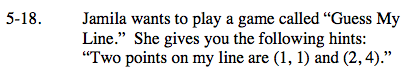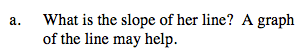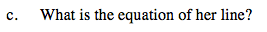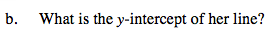Home > AC > Chapter 5 > Lesson 5.1.2 > Problem5-18

5-18.
1.Jamila wants to play a game called “Guess My Line.” She gives you the following hints: “Two points on my line are (1, 1) and (2, 4)." 5-18 HW eTool (Desmos). Homework Help ✎

1. What is the growth rate of her line? A graph of the line may help.

2. What is the y-intercept of her line?

3. What is the equation of her line?Use the rate of growth and the y-intercept to write an equation for the line. y = mx + by-intercept = (0,−2)

Use the eTool below to complete the graph and help solve each part.
Click on the link at right for the full eTool version: AC 5-18 HW eTool